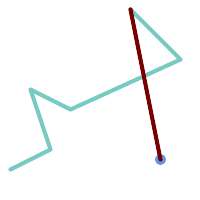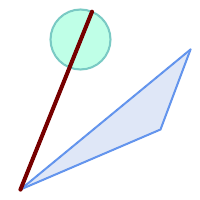## Name

ST_LongestLine — Returns the 2D longest line between two geometries.

## Synopsis

`geometry ST_LongestLine(`geometry g1, geometry g2`)`;

## Description

Returns the 2-dimensional longest line between the points of two geometries. The line returned starts on `g1` and ends on `g2`.

The longest line always occurs between two vertices. The function returns the first longest line if more than one is found. The length of the line is equal to the distance returned by ST_MaxDistance.

If g1 and g2 are the same geometry, returns the line between the two vertices farthest apart in the geometry. This is a diameter of the circle computed by ST_MinimumBoundingCircle

Availability: 1.5.0

## ExamplesLongest line between a point and a line

```SELECT ST_AsText( ST_LongestLine(
'POINT (160 40)',
'LINESTRING (10 30, 50 50, 30 110, 70 90, 180 140, 130 190)' )
) AS lline;
-----------------
LINESTRING(160 40,130 190)
```Longest line between two polygons

```SELECT ST_AsText( ST_LongestLine(
'POLYGON ((190 150, 20 10, 160 70, 190 150))',
ST_Buffer('POINT(80 160)', 30)
) ) AS llinewkt;
-----------------
LINESTRING(20 10,105.3073372946034 186.95518130045156)
```Longest line across a single geometry. The length of the line is equal to the Maximum Distance. The line is a diameter of the Minimum Bounding Circle.

```SELECT ST_AsText( ST_LongestLine( geom, geom)) AS llinewkt,
ST_MaxDistance( geom, geom) AS max_dist,
ST_Length( ST_LongestLine(geom, geom)) AS lenll
FROM (SELECT 'POLYGON ((40 180, 110 160, 180 180, 180 120, 140 90, 160 40, 80 10, 70 40, 20 50, 40 180),
(60 140, 99 77.5, 90 140, 60 140))'::geometry AS geom) AS t;

llinewkt          |      max_dist      |       lenll
---------------------------+--------------------+--------------------
LINESTRING(20 50,180 180) | 206.15528128088303 | 206.15528128088303
```